Practice - Grid Rigid Transformations Terms of Use    Contact Person: Donna Roberts1.
Rectangle ABCD becomes rectangle A'B'C'D' under a translation of 2 units to the left and 4 units down.
a) What are the coordinates of A' ?

Choose:
 (-2,-4) (-4,0) (-4,-2) (-4,-6)

b)
What is the value of B'C' ?
Choose:
 3 units 4 units 2 units 6 units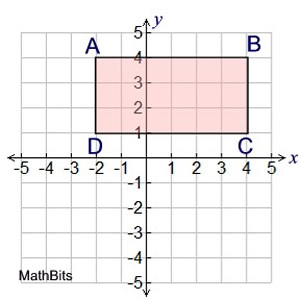2. Seven line segments are shown on the graph below. Which of the segments listed could be the image of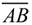after a sequence of reflections, translations, and/or rotations? (Check all that apply, and hit SUBMIT!)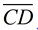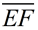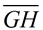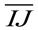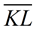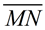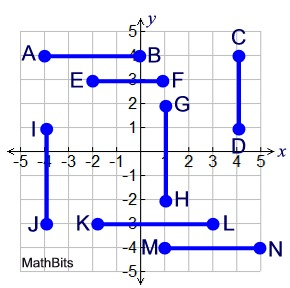3.
ΔABC is shown at the right.
ΔA'B'C' is formed after a rotation 90º counterclockwise about the origin. ΔA''B''C'' is formed after ΔA'B'C' is reflected across the x-axis.

What are the signs of coordinates (x,y) of A'' ?
Choose:
 Both x and y are positive. x is positive, y is negative. x is negative, y is positive. Both x and y are negative.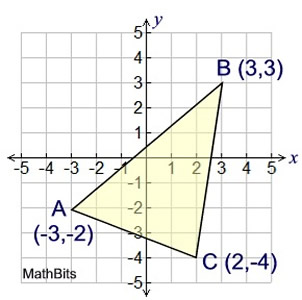4.
The horizontal lines shown on the graph are parallel. Which transformation will show that ∠1 is congruent to ∠2 ?

Choose:
 reflect ∠1 over the x-axis translate ∠1 up and right rotate∠1 180º about (0,0) translate ∠1 down and left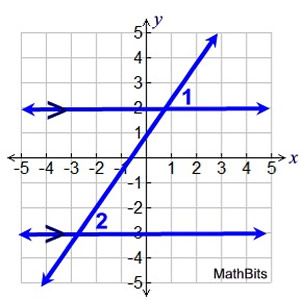5.
Trapezoid ABCD has coordinates
A(-4,5), B(-2,4), C(-2,2) and D(-4,1). After a translation, the image of vertex C is (4,-2). What are the coordinates of the image of vertex D?
Choose:
 (2,-4) (4, 0) (2, 1) (2,-3)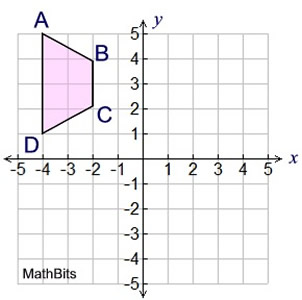6.
The Bolt Soda Company is designing a logo. They start with the figure shown in the third quadrant. They then add the rotation of this figure 90º clockwise about the origin to the design.

What does the new design look like?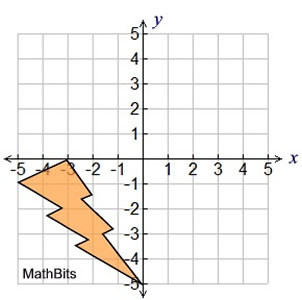Choose: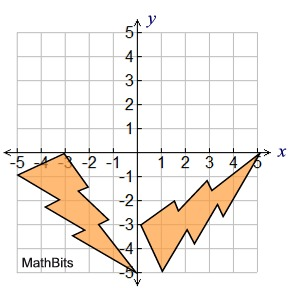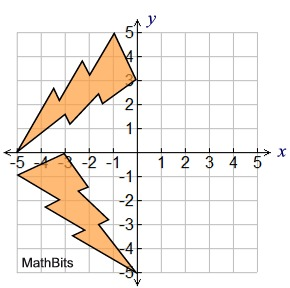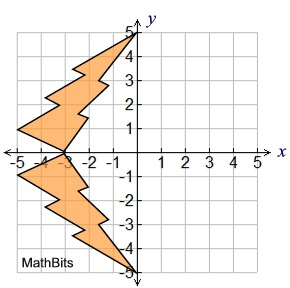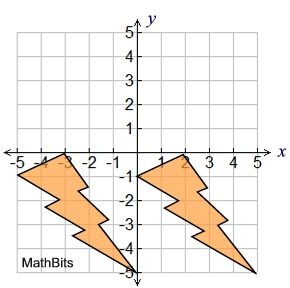7.
Pentagon A is congruent to pentagon B. Which series of transformations can be used to verify this congruence?
Use pentagon A as the pre-image.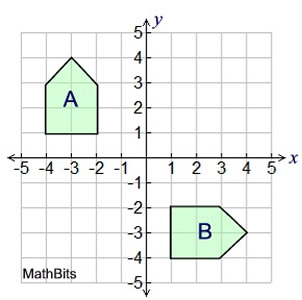Choose:
 rotate 90º clockwise, then reflect over x-axis reflect over x-axis, then reflect over y-axis rotate 90º clockwise, then translate 4 down reflect over x-axis, then translate 4 right

8.
Triangle A is congruent to triangle B. Which of the following transformations cannot transform triangle A to triangle B?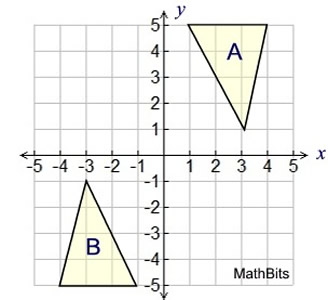Choose:
 reflection in the x-axis, then reflection in the y-axis 180º counterclockwise rotation about the origin reflection in the y-axis, then a translation down of 5 units 180º clockwise rotation about the origin

9.
Rectangle ABCD is congruent to rectangle QRST. Which transformation(s) to ABCD show it is congruent to QRST ?
Choose:
 translate up 6 units and right 1 unit translate left one unit, then reflect over the x-axis translate: (x, y) → (x - 1, y - 6) translate down 2 units and left 1 unit, then reflect over x-axis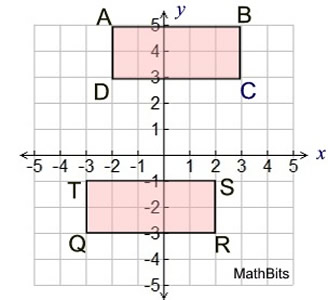10. Triangles A, B and C are congruent.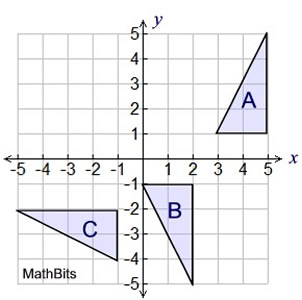a) Which sequence of transformations could verify that triangle A is congruent to triangle B?
Choose:
 reflect over x-axis, then translate 3 units right reflect over x-axis, then translate 3 units left rotate 180º counterclockwise about origin, then translate 2 units left rotate 90º counterclockwise about origin, then translate 2 units left

b)
Which sequence of transformations could verify that triangle A is congruent to triangle C?

Choose:
 rotate 180º clockwise about origin, then translate 3 down rotate 90º counterclockwise about origin, then translate 7 down rotate 180º counterclockwise about origin, then translate 2 units up rotate 90º clockwise about origin, then translate 3 units left